Question

# QUESTION #2 PLEASE 1. Derive the transfer function for the circuit shown below. Plot H(s) versus...

QUESTION #2 PLEASE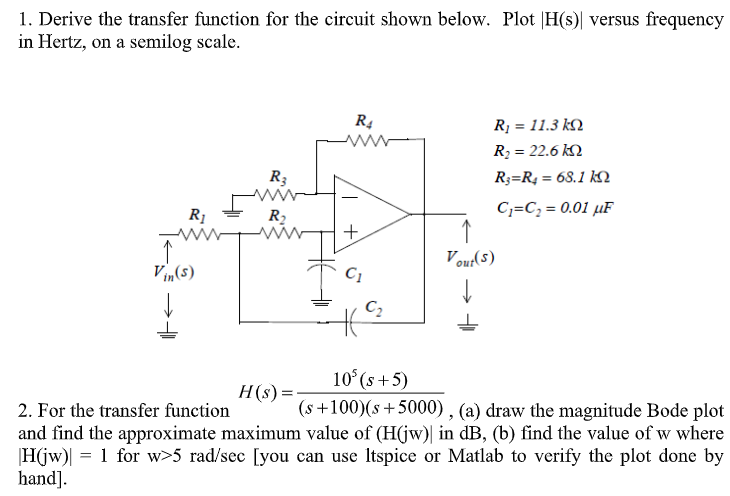1. Derive the transfer function for the circuit shown below. Plot H(s) versus frequency in Hertz, on a semilog scale. Ri 11.3 k Ri 22.6 k R R = 68.1 kN R3 C C 0.01 uF R2 Vout(s) Vin(s) C2 10 (s+5) H(s) = (s+100)(s5000) , (a) draw the magnitude Bode plot 2. For the transfer function and find the approximate maximum value of (H(jw) in dB, (b) find the value of w where 1 for w>5 rad/sec [you can use ltspice or Matlab to verify the plot done by Hjw) hand].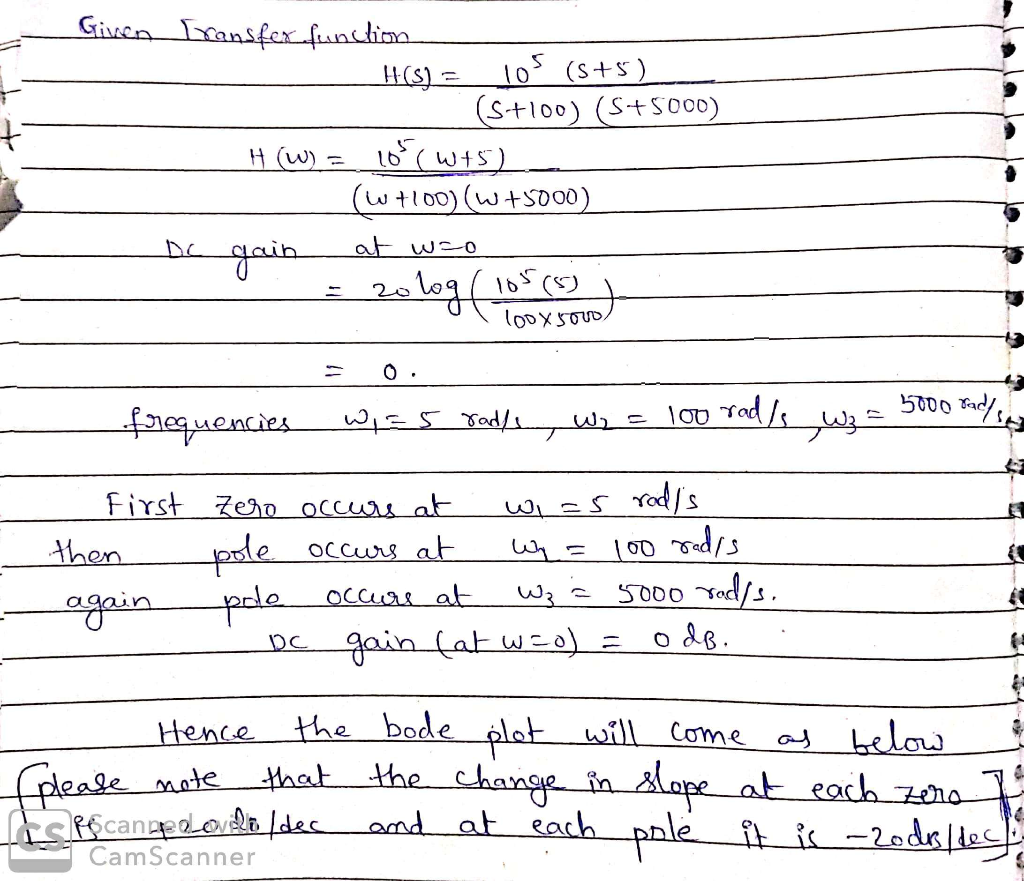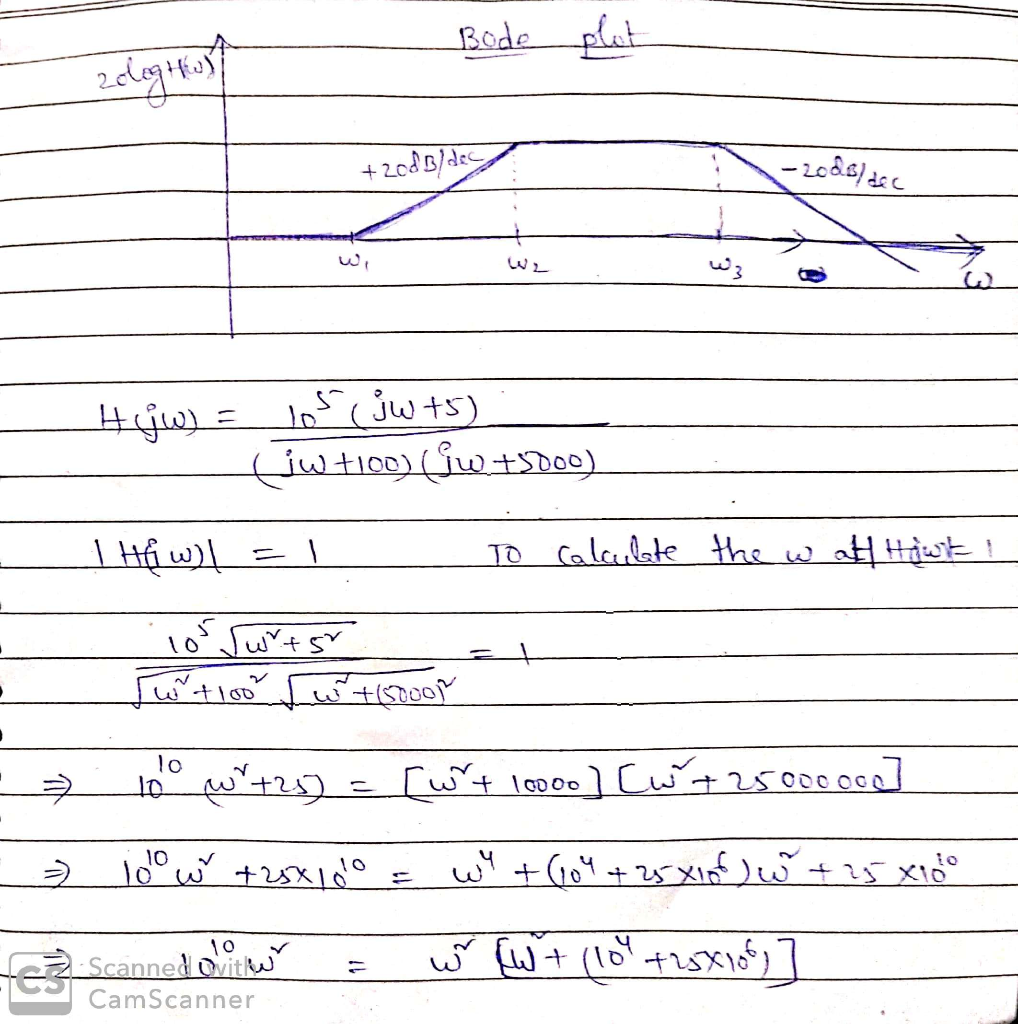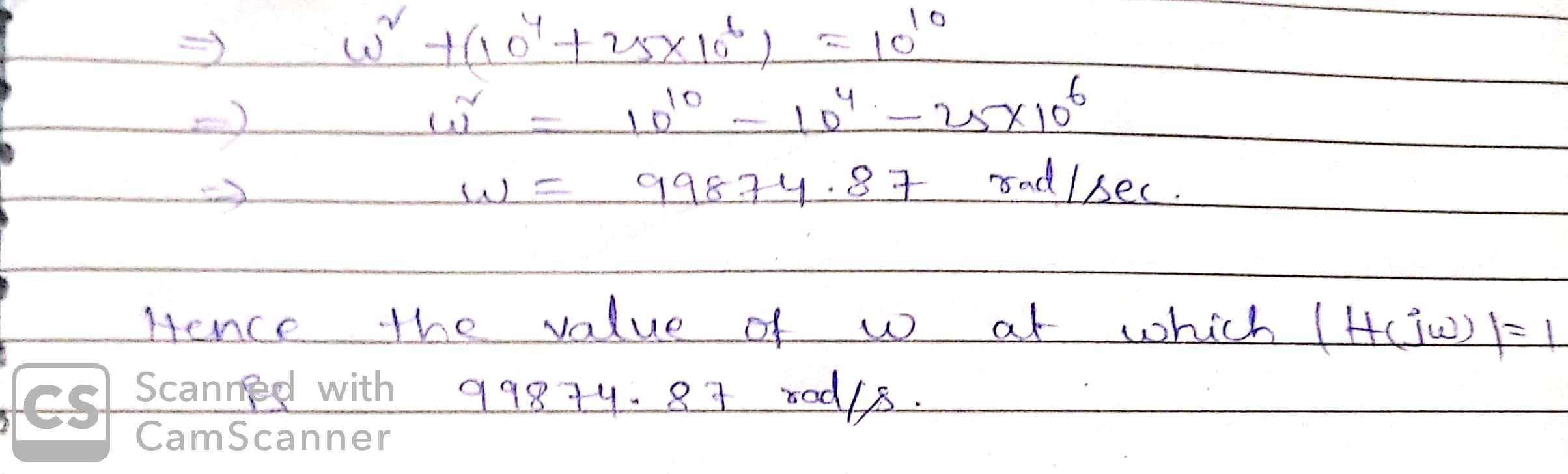#### Earn Coins

Coins can be redeemed for fabulous gifts.

Similar Homework Help Questions
• ### 1. Derive the transfer function for the circuit shown below. Plot FH(s) versus frequency in Hertz,...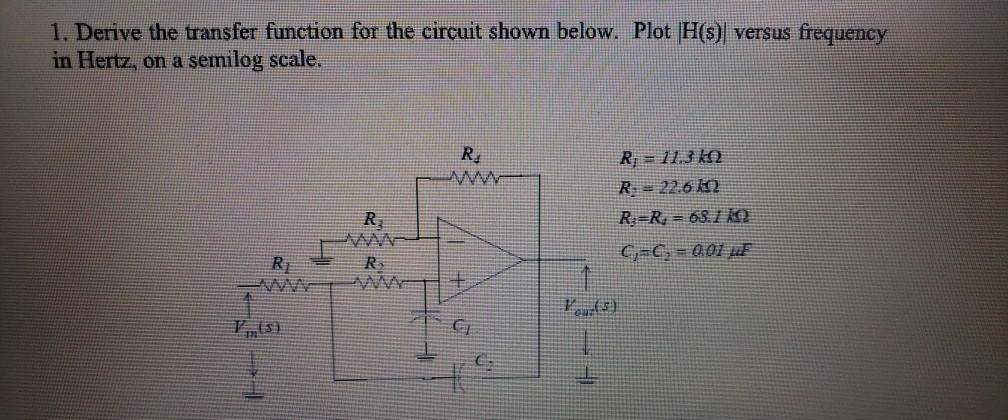1. Derive the transfer function for the circuit shown below. Plot FH(s) versus frequency in Hertz, on a semilog scale. Re R 113 k R 22.6 12 R R 63.12 Rj F)

• ### Problem 2 An RC circuit ( with an active component) has the following transfer function (where...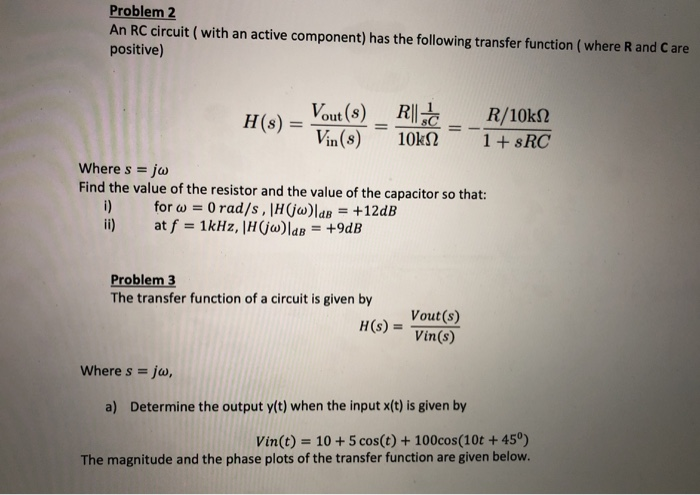Problem 2 An RC circuit ( with an active component) has the following transfer function (where R and Care positive) H(s) - Vout(8) _R|| R/10k12 Vin(8) 10KN 1 + \$RC Where s = jw Find the value of the resistor and the value of the capacitor so that: for w = 0 rad/s, H(jw)lde = +12dB at f = 1kHz, |H(jw)lab = +9dB Problem 3 The transfer function of a circuit is given by H(S) = Vout(s) Vin(s) Where s...

• ### Find the transfer function H(s) for the circuit below. Make s=jw and plot the Bode plot...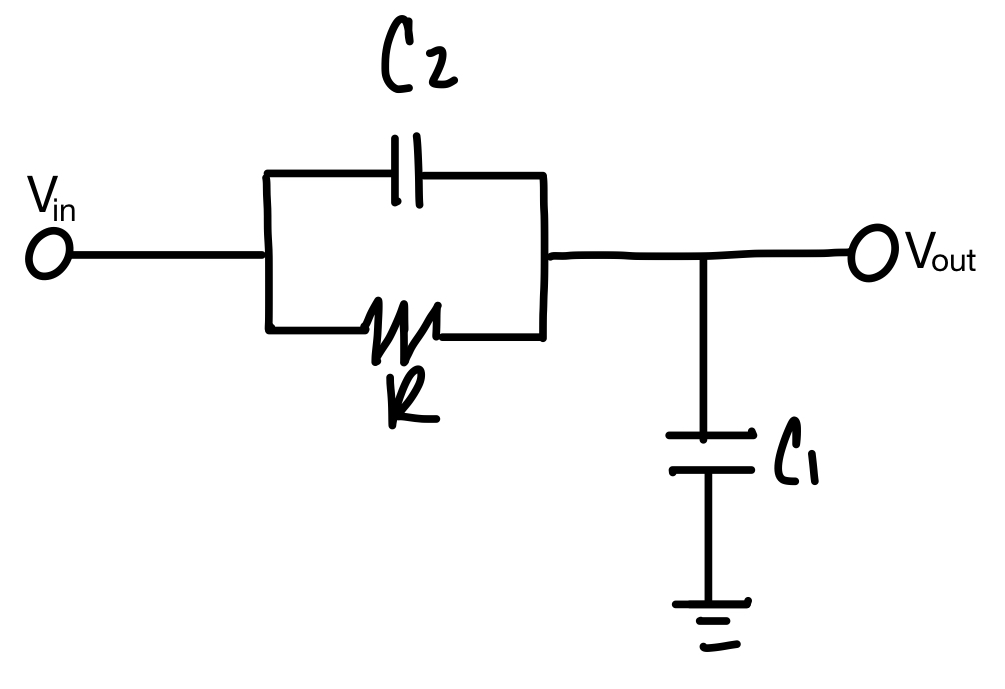Find the transfer function H(s) for the circuit below. Make s=jw and plot the Bode plot (magnitude and phase response) for the transfer function. C = out 페

• ### Q11 (20pts.): For the circuit shown below Derive the transfer function, H(s) assuming the OPAMP's transfer...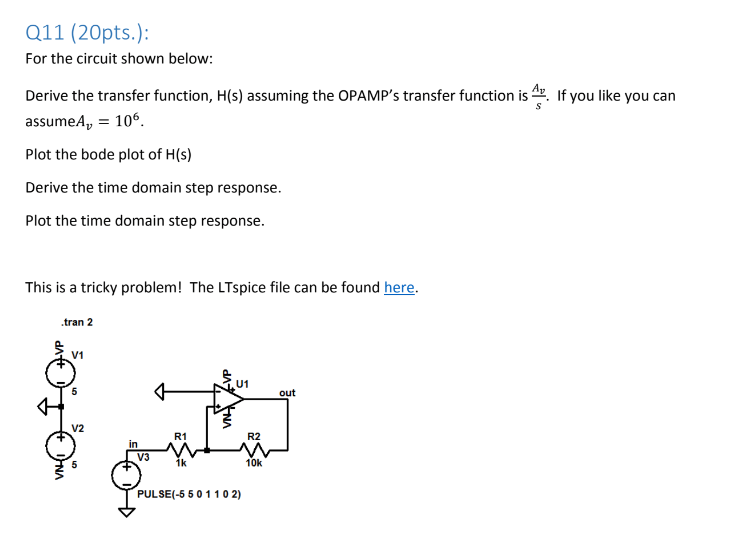Q11 (20pts.): For the circuit shown below Derive the transfer function, H(s) assuming the OPAMP's transfer function is. If you like you can assumeA 106 Plot the bode plot of H(s) Derive the time domain step response. Plot the time domain step response This is a tricky problem! The LTspice file can be found here. .tran 2 V1 U1 out V2 R1 R2 in V3 1k 10k PULSE(-5 50 1102)

• ### Given the circuit below: R3 C1 Vout C2 R1 R3 Vin R2 ts 1 a) derive the transfer function between ...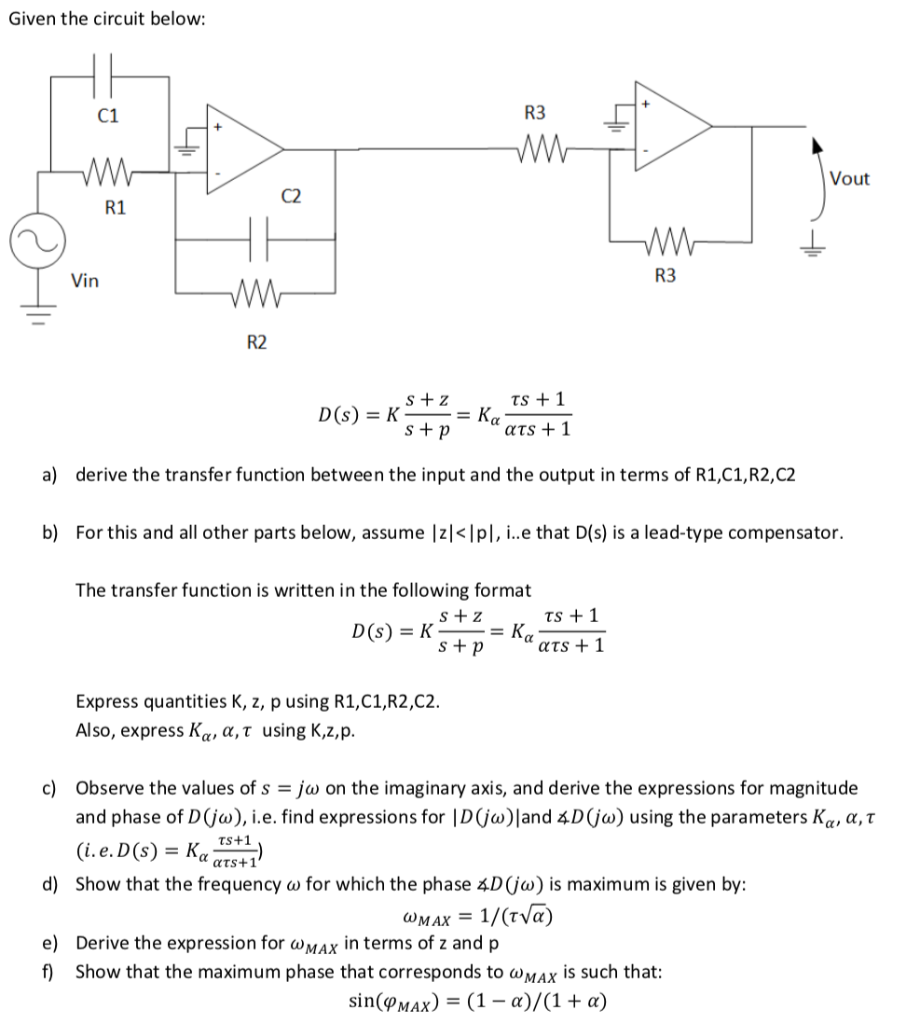Given the circuit below: R3 C1 Vout C2 R1 R3 Vin R2 ts 1 a) derive the transfer function between the input and the output in terms of R1,C1,R2,C2 b) For this and all other parts below, assume Izl<Ipl, i..e that D(s) is a lead-type compensator. The transfer function is written in the following format ts 1 Express quantities K, z, p using R1,C1,R2,C2 Also, express Κα, α, τ using K,z, p c) Observe the values of s-jw on the...

• ### 5. Draw the magnitude characteristic of the Bode plot of the following transfer function: H(s)10(10s +11...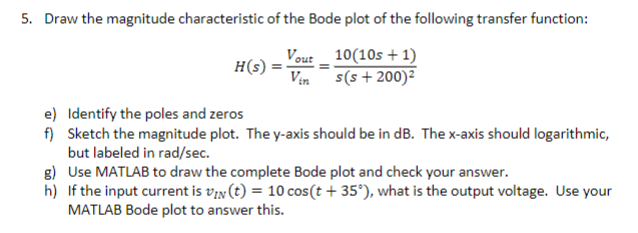5. Draw the magnitude characteristic of the Bode plot of the following transfer function: H(s)10(10s +11 (S + 200)2 Identify the poles and zeros Sketch the magnitude plot. The y-axis should be in dB. The x-axis should logarithmic, but labeled in rad/sec e) f) g) Use MATLAB to draw the complete Bode plot and check your answer. h) If the input current is VIN(t)-10 cos(t+35"),what is the output voltage. Use your MATLAB Bode plot to answer this.

• ### For the circuit shown below (1) Please derive an expression for the transfer function H(jco)- Vout/Vin....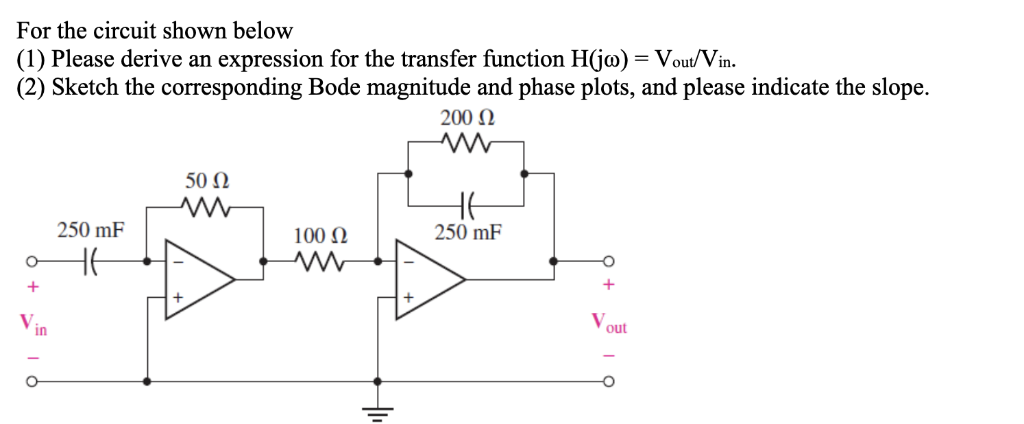For the circuit shown below (1) Please derive an expression for the transfer function H(jco)- Vout/Vin. (2) Sketch the corresponding Bode magnitude and phase plots, and please indicate the slope. 50Ω 250 mF 100 Ω 250 mF in out

• ### -... Find the transfer function H(w) with the Bode magnitude plot shown in Fig. 14.74 H...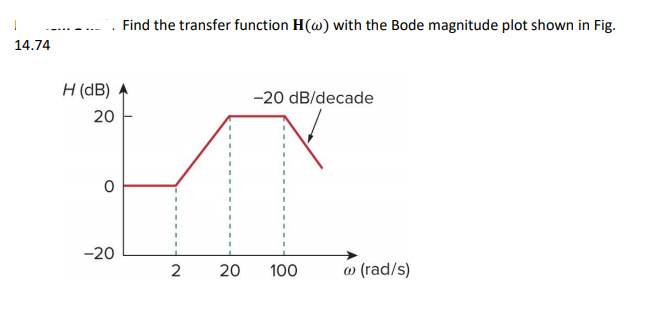-... Find the transfer function H(w) with the Bode magnitude plot shown in Fig. 14.74 H (dB)参 20 20 dB/decade 0 -20L 2 20 100 a) (rad/s)

• ### 4. Sketch the Bode magnitude plot ofa fiter having a transfer function 10's H(s)- (s+500) (s+S0s+...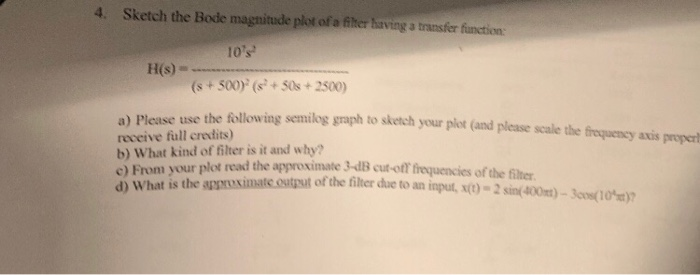4. Sketch the Bode magnitude plot ofa fiter having a transfer function 10's H(s)- (s+500) (s+S0s+2500) he following senilog graph to sketch yo ur plot (and please scale the eequeney axis proper a) Please use t receive full credits) b) What kind of filter is it and why? read the approximate 3-dB cut-off frequencies of the filter From your plot ont is the asrsximate.ostt of the filter due to an input, st)- 2sin(400)-3os(10 a) c) frequency axis properly a) Please...

• ### 4. Draw the magnitude characteristic of the Bode plot of the following transfer function: 10s H...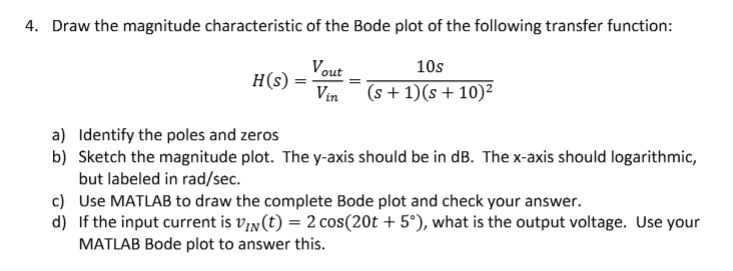4. Draw the magnitude characteristic of the Bode plot of the following transfer function: 10s H (s) =-= (s+1)(s+102 L7n Identify the poles and zeros Sketch the magnitude plot. The y-axis should be in dB. The x-axis should logarithmic, but labeled in rad/sec Use MATLAB to draw the complete Bode plot and check your answer. If the input current is un(t) = 2 cos(20t + 5°), what is the output voltage. Use your MATLAB Bode plot to answer this a)...